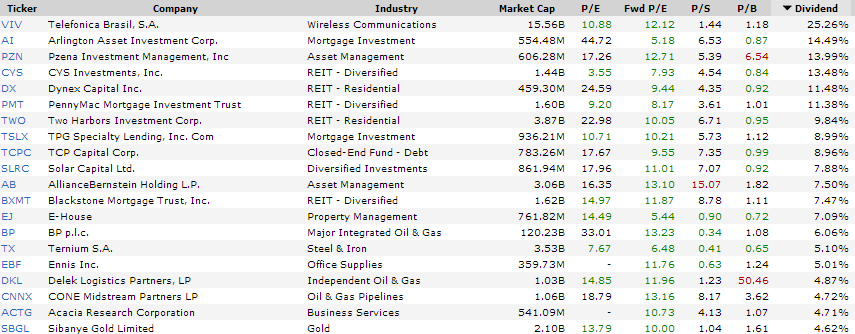## Pages

### 4 Dividend Payer With 10% Yields And Promising Fundamentals

Big dividends are dead? No, I don't think so. Despite the fact that the FED and other national banks killed the interest rates, there are still high and stable dividend payments.

Today I like to focus my thoughts on higher risk stocks with bigger dividends. Those stocks have a really low market capitalization, a high payout ratio and cheap valuation.

As a result, dividend yield ratios explode. Attached is a list of the top 20 results by yield who met my criteria.

These are my criteria in detail:
- Market Cap over \$300 million
- Positive 5Y Earnings Growth Forecast
- Low Forward P/E
- Debt/Equity under 0.5
- Buy Rating from Analysts

These are the 5 top yielding results...

### Telefonica Brasil -- Yield: 3.94%

Telefonica Brasil (NYSE:VIV) employs 18,532 people, generates revenue of \$10,773.20 million and has a net income of \$1,519.53 million. The current market capitalization stands at \$15.56 billion.

Telefonica Brasil’s earnings before interest, taxes, depreciation and amortization (EBITDA) amounts to \$2,255.41 million. The EBITDA margin is 20.94% (the operating margin is 14.62% and the net profit margin 14.10%).

Financials: The total debt represents 10.67% of Telefonica Brasil assets and the total debt in relation to the equity amounts to 17.35%. Due to the financial situation, a return on equity of 11.24% was realized by Telefonica Brasil.

Twelve trailing months earnings per share reached a value of \$1.35. Last fiscal year, Telefonica Brasil paid \$1.49 in the form of dividends to shareholders.

Market Valuation: Here are the price ratios of the company: The P/E ratio is 10.86, the P/S ratio is 1.44 and the P/B ratio is finally 1.19. The dividend yield amounts to 3.94%.

Stock Charts:Long-Term Stock Price Chart of Telefonica Brasil (VIV)Long-Term Dividend Payment History of Telefonica Brasil (VIV)Long-Term Dividend Yield History of Telefonica Brasil (VIV)

### Arlington Asset Investment -- Yield: 14.49%

Arlington Asset Investment (NYSE:AI) employs 11 people, generates revenue of \$84.86 million and has a net income of \$5.95 million. The current market capitalization stands at \$554.65 million.

Arlington Asset Investment’s earnings before interest, taxes, depreciation and amortization (EBITDA) amounts to \$55.45 million. The EBITDA margin is 65.35% (the operating margin is 65.28% and the net profit margin 7.02%).

Financials: The total debt represents 1.00% of Arlington Asset Investment assets and the total debt in relation to the equity amounts to 6.23%. Due to the financial situation, a return on equity of 1.00% was realized by Arlington Asset Investment.

Twelve trailing months earnings per share reached a value of \$0.54. Last fiscal year, Arlington Asset Investment paid \$3.50 in the form of dividends to shareholders.

Market Valuation: Here are the price ratios of the company: The P/E ratio is 45.00, the P/S ratio is 6.54 and the P/B ratio is finally 0.86. The dividend yield amounts to 14.49%.

Stock Charts:Long-Term Stock Price Chart of Arlington Asset Investment (AI)Long-Term Dividend Payment History of Arlington Asset Investment (AI)Long-Term Dividend Yield History of Arlington Asset Investment (AI)

### CYS Investments -- Yield: 13.48%

CYS Investments (NYSE:CYS) employs 16 people, generates revenue of \$317.08 million and has a net income of \$425.61 million. The current market capitalization stands at \$1.44 billion.

CYS Investments’s earnings before interest, taxes, depreciation and amortization (EBITDA) amounts to \$260.37 million. The EBITDA margin is 82.12% (the operating margin is 82.12% and the net profit margin 134.23%).

Financials: The total debt represents 0.00% of CYS Investments assets and the total debt in relation to the equity amounts to 0.00%. Due to the financial situation, a return on equity of 25.13% was realized by CYS Investments.

Twelve trailing months earnings per share reached a value of \$2.51. Last fiscal year, CYS Investments paid \$1.24 in the form of dividends to shareholders.

Market Valuation: Here are the price ratios of the company: The P/E ratio is 3.55, the P/S ratio is 4.54 and the P/B ratio is finally 0.84. The dividend yield amounts to 13.48%.

Stock Charts:Long-Term Stock Price Chart of CYS Investments (CYS)Long-Term Dividend Payment History of CYS Investments (CYS)Long-Term Dividend Yield History of CYS Investments (CYS)

### Dynex Capital -- Yield: 11.48%

Dynex Capital (NYSE:DX) employs 17 people, generates revenue of \$105.64 million and has a net income of \$27.81 million. The current market capitalization stands at \$459.29 million.

Dynex Capital’s earnings before interest, taxes, depreciation and amortization (EBITDA) amounts to \$73.67 million. The EBITDA margin is 69.73% (the operating margin is 26.32% and the net profit margin 26.32%).

Financials: The total debt represents 0.29% of Dynex Capital assets and the total debt in relation to the equity amounts to 1.78%. Due to the financial situation, a return on equity of 3.83% was realized by Dynex Capital.

Twelve trailing months earnings per share reached a value of \$0.34. Last fiscal year, Dynex Capital paid \$1.00 in the form of dividends to shareholders.

Market Valuation: Here are the price ratios of the company: The P/E ratio is 24.57, the P/S ratio is 4.35 and the P/B ratio is finally 0.92. The dividend yield amounts to 11.48%.

Stock Charts:Long-Term Stock Price Chart of Dynex Capital (DX)Long-Term Dividend Payment History of Dynex Capital (DX)Long-Term Dividend Yield History of Dynex Capital (DX)Dividend Payer With High Yields And Promising Fundamentals (click to enlarge),Source: long-term-investments.blogspot.com